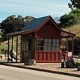# Gas station without pumps

## 2014 April 30

### Loudspeaker analysis

Filed under: Circuits course — gasstationwithoutpumps @ 21:39
Tags: , , , ,

In class today, we analyzed the data that the students collected yesterday in the loudspeaker lab.

I used gnuplot to work through several model fits to the magnitude of impedance as a function of frequency.  The first one was a simple L+R model (though later we got even simpler with just R=8Ω). I showed the difference between fitting Z and fitting$\log(Z)$.

The model clearly was a poor fit, though it has roughly the right shape, with a sort of constant region for a while, then rising impedance with frequency.

I next introduced$(L_{s}||C_{s}||R_{s})$ for the resonance peak, and showed how to fit those parameters without changing R or L for the main model, both by limiting the frequency range and by specifying only the parameters we wanted to optimize.  After fitting the resonance peak, I refit R and L over all the data.  This model did a decent job for lower frequencies, but still had problems at high frequencies.

I then mentioned one hack (that I have played with in the past) that is commonly used to model loudspeakers, of adding more “knees” to the curve to get a wobbly line that tracks the data a little closer.  I did not try to develop this in class, since we have no particular need to stick with standard linear components.

Instead I introduced them to a non-linear inductor, whose impedance is$j \omega^{\alpha} L$ instead of$j \omega L$, and fit that after fitting the resonance peak.  (This is the same model I developed in Better model for loudspeaker, though the parameterization may be slightly different.)

Here is the plot we produced (note that it was not properly labeled—I expect the students to be able to do that themselves by now):Final fit developed in class today. I’m pretty happy with how close a fit we get with only 6 parameters.

I also mentioned the concept of a semi-inductor (the formula above with$\alpha=0.5$), and showed them how that fit (OK, but not as good as$\alpha=0.627$).

The model-fitting took a little longer than I had expected.  I’d wanted to spend 45 minutes on it, but spent more like 55.  I think that the lessons on how to fit parameters when they affect just part of the curve (as for the resonance peak), and the basic lesson that there are no true models, just more or less useful ones, were worth the time, though. We talked a little about when each of the models we used might be useful.

The R=8Ω model came up as being a useful one for signals between 200Hz and 10kHz—most of the audio range of interest for speech, but not so useful near the resonance peak or for high frequency.  I pointed out that we’d want to know the characteristics of the loudspeaker at high frequency later this quarter for the class-D power amp lab, which was why I went so far as to introduce non-textbook non-linear devices like the generalized inductor.

In the last 15 minutes of the class, I managed to talk a little about discrete values and discrete time, explaining what made a 16-bit ADC 16 bits, and emphasizing again the distinction between resolution, repeatability, and accuracy (resolution is 1LSB, repeatability for the ADC converters on the board we are using is about ±4LSB, and the accuracy is about ±3%, though the accuracy can be improved by external measurement of the reference voltage).

I only briefly mentioned sampling and aliasing, which they will be examining in tomorrow’s lab.  I’m hoping they read the handout, as that has some of the explanation missing from today’s lecture.  I’m still trying to decide whether to bring the stroboscope into lab as a demo.

Despite not covering aliasing and the Nyquist theorem today, I was pretty happy with how the class went.

Here is the gnuplot script developed in class today (it was all typed live in class without notes—hence the lack of comments and other niceties). Code was modified in place as we went through the different models, so many of the initial fits are not shown here.

j=sqrt(-1)

zc(f,c) = 1/(j*2*pi*f*c)
zl(f,l) = j*2*pi*f*l

zsemi(f,l,alpha) = j * (2*pi*f)**alpha *l

zpar(z1,z2) = z1*z2/(z1+z2)  # parallel impedances

rlrlc(f, R, L, rs, ls, cs) = abs(R + zl(f,L) + zpar(zl(f,ls), zpar(zc(f,cs), rs)))
rlarlc(f, R, L, alpha, rs, ls, cs) = abs(R + zsemi(f,L,alpha) + zpar(zl(f,ls), zpar(zc(f,cs), rs)))

set logscale xy

R=8
L=10e-3
alpha=0.5

rs=15
ls =1e-3
cs = 1e-3

fit [50:500] log(rlarlc(x,R,L,alpha,rs,ls,cs)) "10W-loudspeaker-47ohm-more.data" using 1:(log($3/$2 *Rload)) via rs,ls,cs

fit log(rlarlc(x,R,L,alpha,rs,ls,cs)) "10W-loudspeaker-47ohm-more.data" using 1:(log($3/$2 *Rload)) via R,L,alpha,rs

set samples 1000

plot "10W-loudspeaker-47ohm-more.data" using 1:($3/$2 *Rload) notitle, \
rlarlc(x,R,L,alpha,rs,ls,cs)  title sprintf("%.3f ohm + %.3eH (^%.3f) + (%.3fohm || %.2fmH || %.2fuF)", R, L, alpha, rs, ls*1e3,cs*1e6)


## 2 Comments »

1.[…] the plot above with the hand-measured ones at Better model for loudspeaker or Loudspeaker analysis.  We can see the limitations of the model even more clearly when we look at the phase […]

Pingback by Loudspeaker impedance with Analog Discovery 2 | Gas station without pumps — 2016 December 13 @ 23:12

2.[…] and an 8Ω oval loudspeaker that we used to use in the class until Parts Express sold out of them (Loudspeaker analysis, Better model for loudspeaker). I only collected data out to 1MHz, since my previous experience […]

Pingback by Changing the loudspeaker lab | Gas station without pumps — 2017 June 28 @ 21:38

This site uses Akismet to reduce spam. Learn how your comment data is processed.